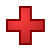Games
Problems
Go Pro!

# Algebraic Fractions

Reference > Mathematics > AlgebraAn introduction to algebraic fractions, and an overview of basic operations with non-algebraic fractionsHow to multiply algebraic fractions, with a review of multiplying numeric fractionsRules for dividing algebraic fractions, with examples of both numeric and non-numeric fractionsHow to add algebraic fractions by finding a least common denominatorOne way of looking at fraction subtraction problems is to rewrite them as addition problemsFractions within fractions - how to simplify more complicated rational expressionsHow to solve equations with rational expressions - clearing equations of algebraic fractions# Blogs on This SiteReviews and book lists - books we love!The site administrator fields questions from visitors.Like us on Facebook to get updates about new resources